# COUNTIF Function

Counts the number of cells that meet a specific criterion

## What is the COUNTIF Function?

The COUNTIF function will count the number of cells that meet a specific criterion. The function is categorized under Excel Statistical functions.

In financial analysis, the COUNTIF function is quite helpful. For example, when we want to count the number of times a salesperson exceeded their target, we can do this by using COUNTIF.

### Formula

=COUNTIF(Range, criteria)

The COUNTIF function uses the following arguments:

1. Range (required argument) – This defines one or several cells that we wish to count. The range of cells are those cells that will be tested against the given criteria and counted if the criteria are satisfied.
2. Criteria – This is a condition defined by us. It is tested against each of the cells in the supplied range.

The given criteria can be any of the following:

1. A numeric value. It can be an integer, decimal, date, time, or logical value.
2. A text string. It may include wildcards. Wildcards can be a ? (question mark) or an * (asterisk). A ? matches any single character, whereas, * matches any sequence of characters. If we wish to actually find the ? (question mark) or * (asterisk) character, we need to type the ~ symbol before this character in our search.

### How to use the COUNTIF Function in Excel?

As a worksheet function, the COUNTIF Function can be entered as part of a formula in a cell of a worksheet. To understand the uses of the function, let us consider a few examples:

#### Example 1

Suppose we are given a list of employees who exceeded sales targets and won the Best Salesperson award for the year. Using COUNTIF, we can find out which person won the highest number of awards.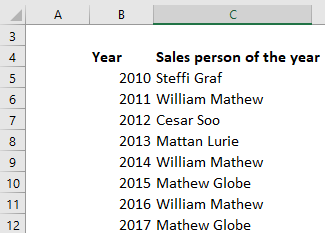The formula to use would be =COUNT(C5:C12, C5) to get the maximum count, as shown below: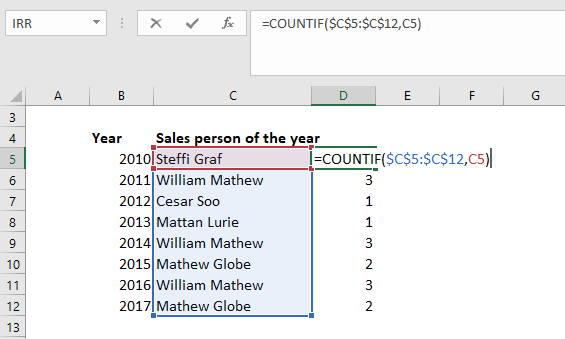We will now drag the formula for all salespersons and get the results below: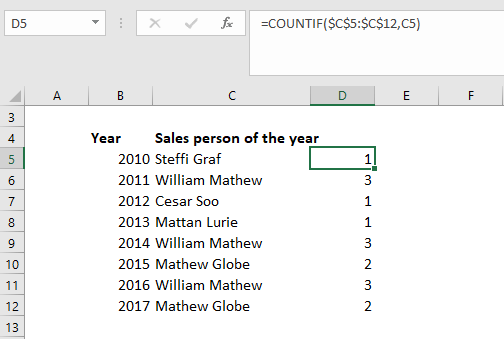As seen above, the name William Mathew appeared three times. Remember, a criterion is case insensitive. Even if we type “william mathew” as the criterion in the above formula, it will produce the same result.

#### Example 2

Let’s see how this function behaves when we deal with numbers. The function works for numbers as efficiently as it does for text values. Suppose we are given the following data: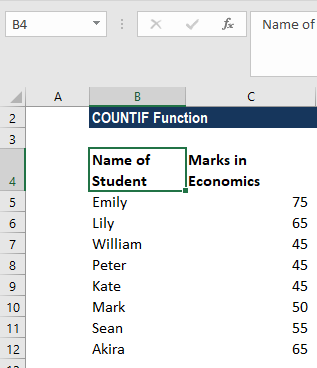Using the formula =COUNTIF(C5:C12,C5), we can see that the function works well for numbers, too.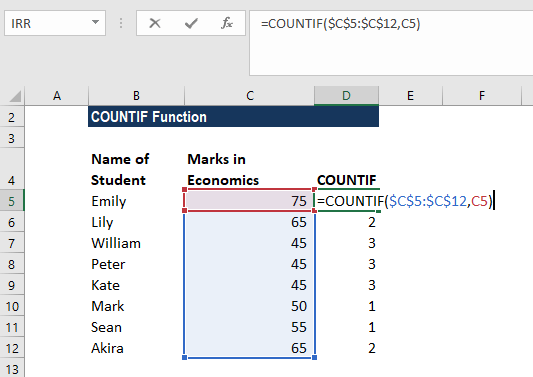The results we get are shown below: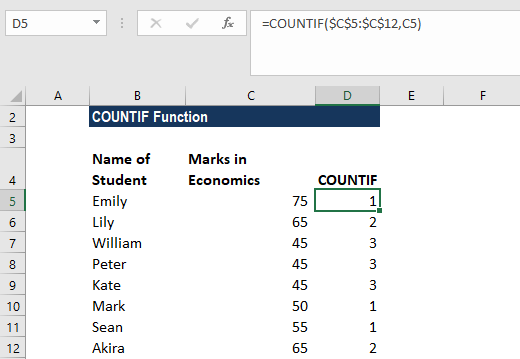It shows that 3 students scored 45 in Economics.

#### Example 3

Let’s see how wildcards can be used with COUNTIF function. Suppose we employ several project managers who handle different projects. We wish to know how many projects are being handled by a particular manager. In such a scenario, we can use the COUNTIF function.

We are given the following data: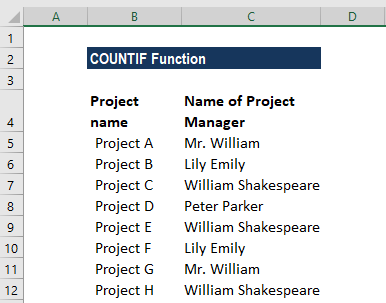The name of Project Manager William Shakespeare is written in several ways. So we would enter “*William*” as the search criteria =COUNTIF(C5:C12, “*William*”).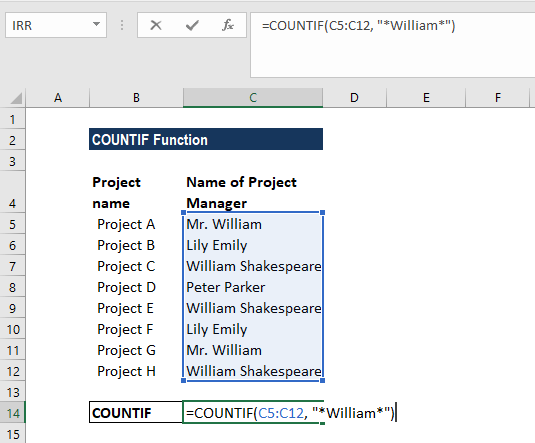The results are as follows: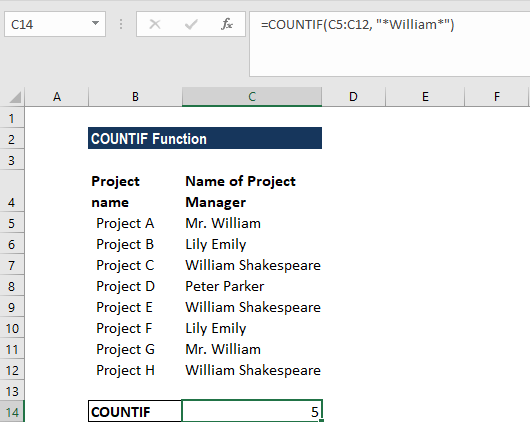If we need to match any single character, we need to enter a question mark instead of an asterisk.

### Some notes about the COUNTIF Function:

1. The COUNTIF function is available from MS Excel 2000.
2. #VALUE! error – Occurs if the given criteria argument is a text string that is greater than 255 characters in length.

Thanks for reading CFI’s guide to important Excel functions! By taking the time to learn and master these functions, you’ll significantly speed up your financial analysis. To learn more, check out these additional CFI resources:

• Excel Functions for Finance